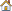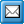﻿ Formula Examples
QPR Knowledge Base 2017.1

Formula ExamplesMetrics User's Guide > Using QPR Metrics > Formulas:

Formula ExamplesFormula ExamplesThis section contains a list of examples to demonstrate the common uses of various parameters and built-in functions in formulas. For illustrated examples that demonstrate how formulas work in a scorecard, go to How Formulas Work in Practice.

To calculate the value for an element with the element identifier "Mea1", with series "Alarm Value" (series identifier ALA):

MEA1.ALA()

To calculate a doubled value for an element with the element identifier "Mea1", with series "Alarm Value" (series identifier ALA):

MEA1.ALA()*2

To calculate the value for an element by taking the sum of the series "Actual Value" (series identifier "ACT") of the three elements Mea5, Mea6, and Mea7:

SUM(MEA5.ACT(), MEA6.ACT(),MEA7.ACT())

To calculate the range value for an element with the element identifier "Mea2", with series "Actual Value" (series identifier ACT):

RANGE(MEA2.ACT())

To calculate the range value from the previous period of an element with the element identifier "Mea2", with series "Actual Value" (series identifier "ACT"):

RANGE(MEA2.ACT(-1))

To calculate the range value from the latest period of an element with the element identifier "Mea2", with series "Actual Value" (series identifier "ACT"), :

RANGE(MEA2.ACT(latest))

To get a value of the default series of an element with the element identifier "Mea1"

MEA1._DEFAULT()

To get the values of the default series from every child element:

SUM(?._DEFAULT())

The average of all the sub-elements of the current element, with the default series:

AVERAGE(?._DEFAULT())

To calculate the average of the latest range values of all the sub-elements of the current element:

AVERAGE(RANGE(?._DEFAULT(latest)))

To calculate the average of the latest values of all the sub-element's series "Actual Value" (series identifier ACT), while ignoring null values:

AVERAGE_(?.ACT(latest))

To calculate the maximum of the latest values of all the sub-element's series "Target Value" (series identifier TAR):

MAX(?.TAR(latest))

To calculate the value of an element by taking average of the series "Forecasted Value" (series identifier "FOR") of the element "Mea1" from the past three periods:

AVERAGE(MEA1.FOR(-1), MEA1.FOR(-2), MEA1.FOR(-3))

To take the maximum value of all the child elements' series "Target Value" (series identifier TAR) and sum it with the series "Actual Value" (series identifier "ACT") of the element identifier "Mea1" and the series "Actual Value" (series identifier "ACT") of the element identifier "Mea2":

SUM(MEA1.ACT(),MEA2.ACT(), MAX(?.TAR()))

To get an average from the latest values of the series "Actual Value" (series identifier ACT) and the previous period's value for the element identifier "Mea32", with series "Actual Value" (series identifier "ACT"):

AVERAGE(?.ACT(latest),MEA32.ACT(-1))

To calculate weighted averages: In the Value settings, define a specific series for each measure that contains the weighting coefficients. Define the identifier of the series to be e.g. WW. Now in a parent element you can calculate the weighted average of all the subelements with the formula:

SUM(?.ACT()*?.WW())/SUM(?.WW())